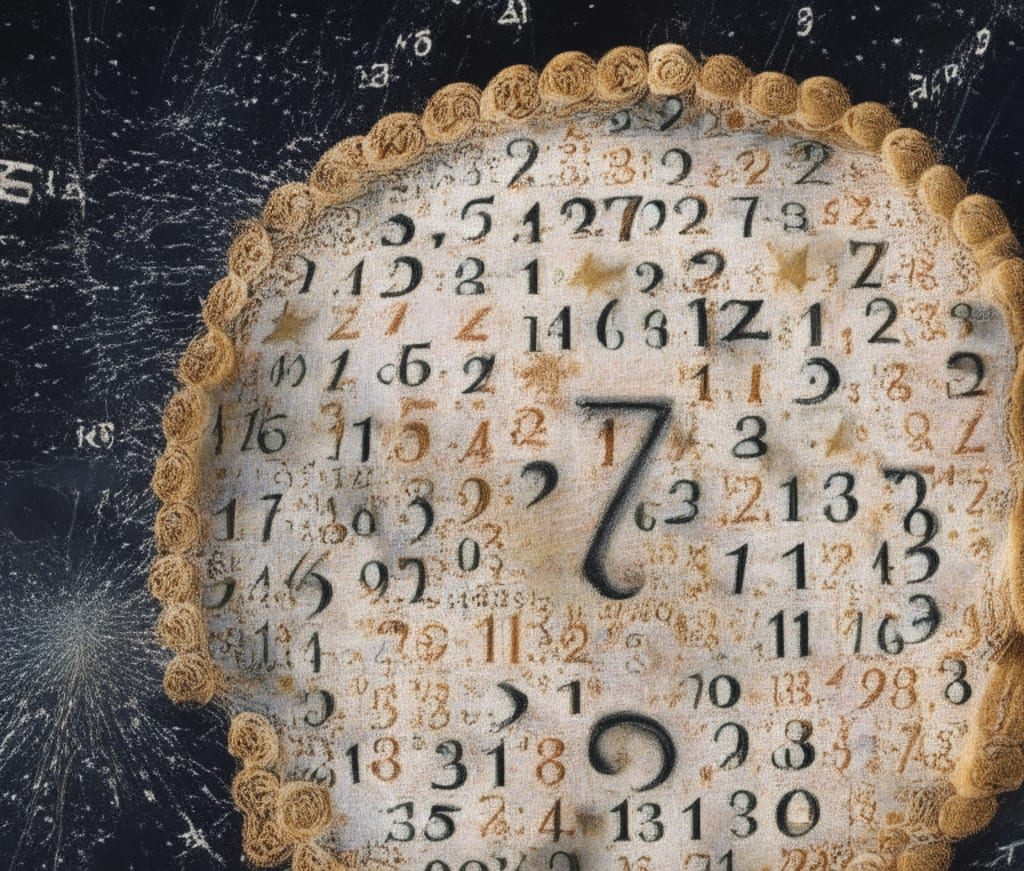# Unveiling the Mysteries of Pi

## How Scientists Push the Boundaries of Calculation

By Panagiotis Published 4 months ago 3 min read
LikeIntroduction:

Pi (π) is an extraordinary mathematical constant that has fascinated and intrigued scientists, mathematicians, and enthusiasts for centuries. Its infinite and non-repeating decimal representation has made it a subject of ongoing exploration. While the value of pi has been known for thousands of years, the quest to calculate more and more digits of this irrational number continues to captivate the scientific community. In this article, we delve into the world of pi and explore how scientists manage to compute increasingly precise values of this enigmatic constant.

Understanding Pi:

Pi represents the ratio of a circle's circumference to its diameter and is often approximated as 3.14159. However, its decimal expansion extends infinitely without a repeating pattern, making it a transcendental number. This unique property of pi has sparked mathematical curiosity and propelled the development of sophisticated algorithms and computational techniques to uncover its deeper intricacies.

Historical Pursuit of Pi:

Throughout history, mathematicians have strived to calculate pi to as many decimal places as possible. In ancient times, Archimedes used a geometric approach to estimate pi, while later mathematicians like Isaac Newton and John Machin developed formulae and algorithms to calculate its value. However, manually computing pi to a high degree of accuracy was a daunting and time-consuming task.

Computers and Pi Calculation:

The advent of modern computers revolutionized pi calculation. With their immense computational power, scientists could explore new avenues for approximating pi. The most common method used today is the iterative algorithms, such as the Bailey-Borwein-Plouffe (BBP) formula, the Chudnovsky algorithm, and the Gauss-Legendre algorithm. These algorithms exploit mathematical series and formulas to compute pi to millions, billions, or even trillions of decimal places.

Supercomputers and Distributed Computing:

As the race to calculate more digits of pi intensifies, scientists harness the power of supercomputers and distributed computing networks. Supercomputers, with their massive parallel processing capabilities, can perform complex calculations simultaneously, significantly speeding up the process. Furthermore, projects like the "Pi Hex" and "Pi in the Sky" employ distributed computing networks, allowing individuals worldwide to contribute their idle computer processing power to compute additional digits of pi collectively.

Record-breaking Achievements:

Thanks to these advancements, remarkable progress has been made in calculating pi to mind-boggling precision. In recent years, records have been shattered repeatedly. In 2019, Emma Haruka Iwao, a Google employee, used the power of the cloud to calculate pi to a staggering 31.4 trillion (31,415,926,535,897) decimal places, setting a new world record. Such achievements not only showcase the capabilities of modern computing but also highlight humanity's relentless pursuit of mathematical knowledge.

The Practical Significance of Calculating More Digits:

One may wonder about the practical implications of calculating billions or trillions of decimal places of pi. While the vast majority of these digits hold little direct practical value, they serve as a testament to the power of human ingenuity and our ability to push the boundaries of knowledge. Additionally, pi finds applications in various fields such as physics, engineering, and cryptography. In these domains, the availability of more precise values of pi can contribute to improved accuracy and efficiency in calculations.

Conclusion:

The quest to calculate more digits of pi represents an ongoing scientific endeavor that intertwines mathematics, computer science, and the human thirst for knowledge. With each new breakthrough in algorithmic development and computational power, scientists inch closer to uncovering the mysteries of pi's infinite decimal expansion. While the practical applications may be limited, the pursuit of pi stands as a symbol of our unyielding curiosity and determination to explore the deepest recesses of the mathematical universe.

how to
Like

How does it work?

There are no comments for this story

Be the first to respond and start the conversation.